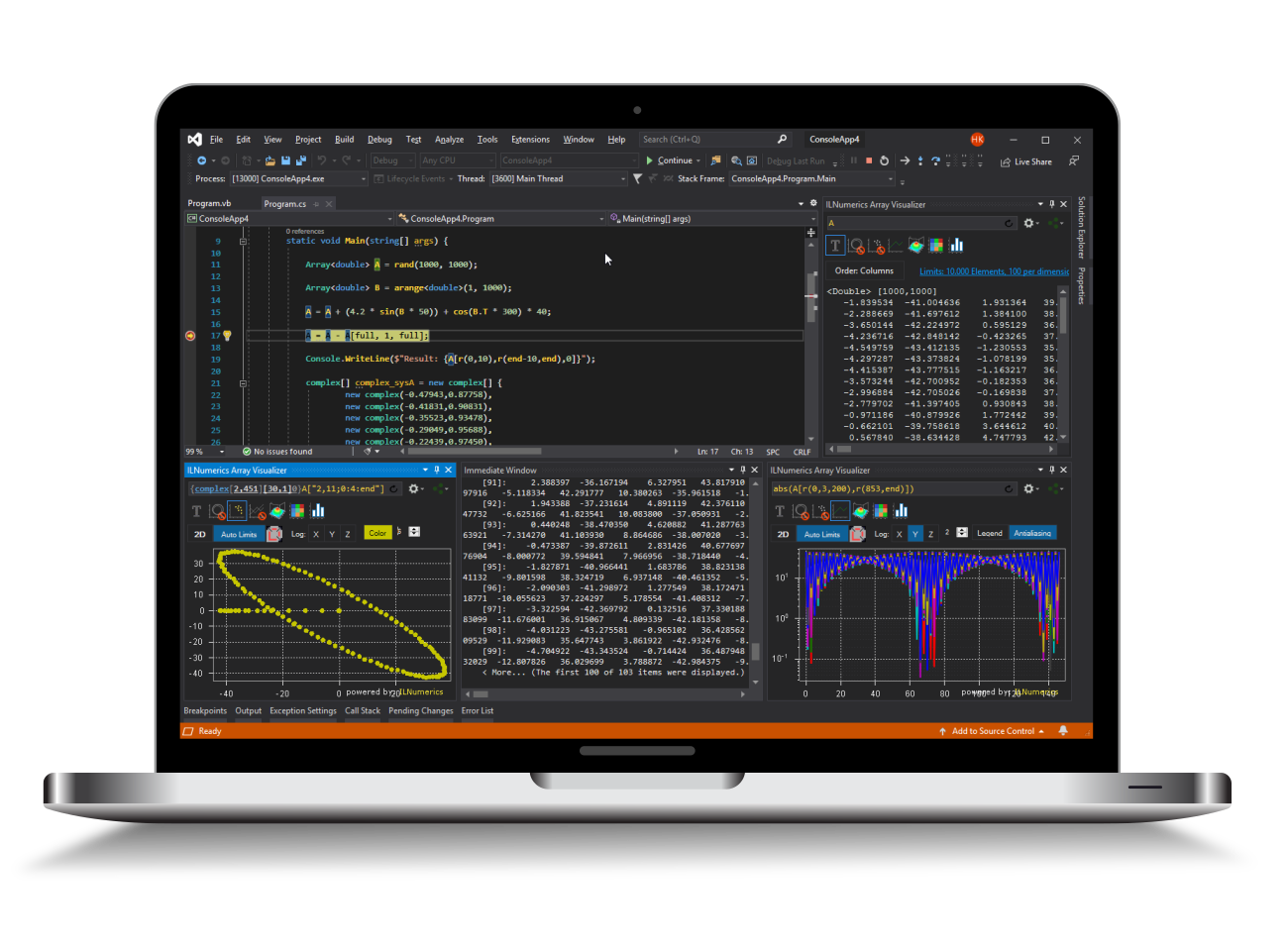Industrial Data Science
in C# and .NET:
Simple. Fast. Reliable.ILNumerics - Technical Computing

Modern High Performance Tools for Technical

Computing and Visualization in Industry and Science

tgt

# LU decomposition for .NET (C# and Visual Basic)

The lu decomposition factorizes a real or complex matrix $A$ into a lower triangular matrix $L$ and an upper triangular matrix $U$.

$$A = L \cdot U$$

Unlike the QR decomposition and the singular value decomposition (SVD) the LU composition only works for square matrices $A$.

## Using the LU decomposition in .NET (C# and Visual Basic)

The matrices $L$ and $U$ are such that they fulfill the equation

$$A = L \cdot U$$

To this end the rows of $L$ have been permuted within the routine. If you want the unpermuted matrix $L$ you can use an overload